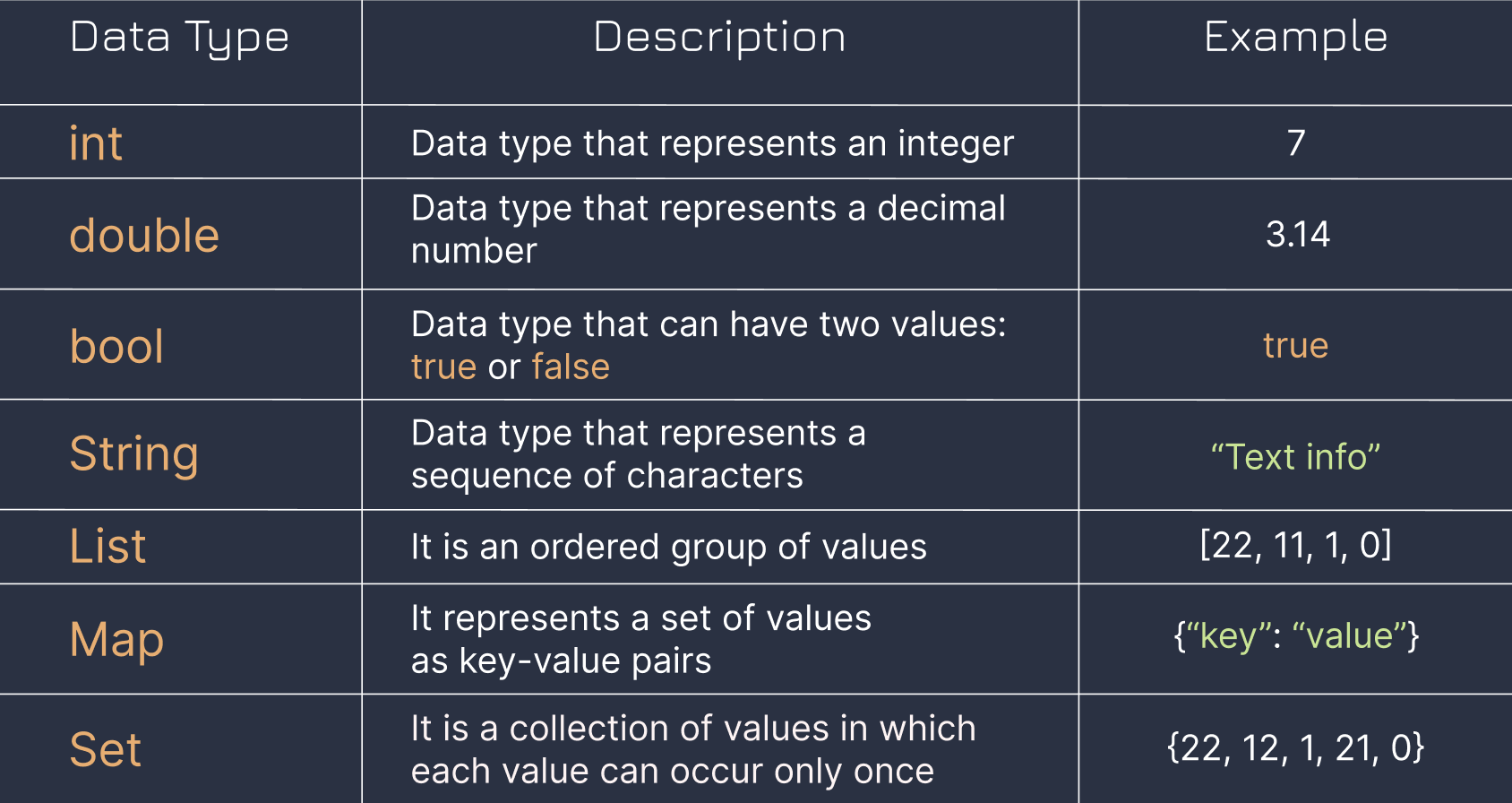Course Content

# Introduction to Dart

1. First Acquaintance with Dart

2. Variables and data types

3. Conditional statements

4. List and String

Introduction to Dart

## What are data types?

Dart supports different data types for different tasks. Like in life, we use words to communicate and numbers to calculate.

• Dart allows us to use numbers for mathematical operations:main.dart• In Dart, we can join strings:main.dart• We can use the `bool` data type to print whether a statement is `true` or `false`:main.dartIn the future, we will learn to work with all types of data, and in the following chapters we will consider the simplest of them.

## What are the differences between data types?For example, we can perform mathematical operations between `int` and `double` because both are numbers.

### Note

`int` and `double` are numbers, but the `int` data type does not store decimals, so it can only store integers. But the `double` can store any number.

However, we cannot add a `String` to an `int` or `double` because a `String` is simply a sequence of characters, which does not support mathematical operations.main.dart